• matlab缩放函数图像代码数字可缩放矢量图形（SVG）导出 将2D和3D Matlab图转换为可缩放矢量格式（SVG）。 此格式由W3C（）指定，可以使用Internet浏览器查看和打印。 添加了对过滤器，剪切和刻度标记扩展的初步支持...
• matlab缩放函数图像代码单发MultiBox检测器 注意：此存储库不再维护。 对于主动维护的易于使用的物体检测器，我建议考虑使用PyTorch Mask R-CNN。 该目录包含用于训练和评估本文所述的SSD对象检测器的代码： SSD: ...
• matlab缩放函数图像代码清除 评估多目标跟踪算法的标准度量标准是CLEAR MOT。 该度量标准在论文中进行了描述。 使用我们的代码的论文  D. Karatzas，F。Shafait，S。Uchida，M。Iwamura，L。Gomez i Bigorda，...
• Write a function Reduce / Expand that has an image as an input and the output should be re-sampled copy of half / twice the width and height of the input image.
• matlab开发-使用相邻插值缩放动画。最近邻插值用于将图像缩放任意
• 我们经常有对图像缩放的要求，这方面有很多现成的代码和函数，比如matlab中的resize,但是在视频分析中有时候将不同长度的视频统一的缩放到指定长度帧数，本文提供了将视频缩放到指定长度帧的MATLAB 代码。...

我们经常有对图像缩放的要求，这方面有很多现成的代码和函数，比如matlab中的resize,但是在视频分析中有时候将不同长度的视频统一的缩放到指定长度帧数，本文提供了将视频缩放到指定长度帧的MATLAB 代码。

function out = video2_100( video,aim,length)
%VIDEO2_100 此处显示有关此函数的摘要
%   此处显示详细说明
%参数含义：video:输入视频的路径：如D:test.avi
% aim:输出视频路径：如D:out.avi
%length:缩放到的帧数
index=0;
n=3;
numFrames = obj.NumberOfFrames; % 帧的总数  NumberOfFrames
step=numFrames/100;
[height,width,~]=size(frame);

temp_video=zeros(height,width,3,100);
video=zeros(height,width,3,numFrames-1);
size(temp_video)

for k = 1 :1: numFrames-1% 读取所有帧
index=index+1;
video(:,:,:,k)=frame;
%       frame_name=strcat('5_',num2str(index,'%06d'),'.jpg');
%     frame_path=fullfile('E:\pose-estimation\三中主校\Images',frame_name);
%    % imshow(frame);%显示帧
%       imwrite(frame,frame_path,'jpg');% 保存帧
end
%  imshow(uint8(video(:,:,:,1)));
%方法一：求每个像素点亮度关于时间的函数

%  for i=1:3
%      for j=1:height
%          j
%          for k=1:width
%              x=(1:numFrames-1);
%              y=video(j,k,i,:);
%              y=reshape(y,1,numFrames-1);
%              y1=polyfit(x,y,n);
%             for o=1:100
%
%                 temp_video(j,k,i,o)=y1(1)*((o-1)*step)^3+y1(2)*((o-1)*step)^2+y1(3)*((o-1)*step)^1+y1(4)*((o-1)*step)^0;
%             end
%
%          end
%      end
%  end
%

%方法二：最近邻插值
for i=1:3
for j=1:height

for k=1:width
for m=1:length
temp_video(j,k,i,m)=video(j,k,i,round(m/length*(numFrames-1)));
end

end
end
end
%  imshow(uint8(temp_video(:,:,:,1)));
fps = 25; %帧率

if(exist(aim,'file'))
delete aim
end

%生成视频的参数设定
aviobj=VideoWriter(aim);  %创建一个avi视频文件对象，开始时其为空
aviobj.FrameRate=fps;

open(aviobj);%Open file for writing video data
for i=1：size(temp_video,4)
frame=temp_video(:,:,:,i)
writeVideo(aviobj,frame);
end

close(aviobj);

end

展开全文• 许多常用的命令行选项可用于操作中心轴，以及从图形的菜单栏中缩放和平移。 有关功能的更完整描述和演示，请参阅帮助。 此函数依赖于未记录和不受支持的属性，因此它可能不适用于某些版本的 Matlab。 在 2007a、...matlab
• 包含最近邻插值、双线性插值和三次插值的matlab代码，自己已经测试过，使用的时候注意将图片转换为位图，否则会出现错误。
• 按峰值缩放语音 介绍视频： https://youtu.be/ZvVc9eKUxgo 介绍https://medium.com/audio-processing-by-matlab/audio-normalization-by-matlab-6407c69dbea 教程和联系 Jarvus ...matlab
•matlab
• 使用临近插值法和双线性插值法进行图像处理，将彩色图像缩放之后仍输出彩色图像。
• 这里介绍matlab gui设计之缩放，实现任意控件的缩放和不缩放设计 这里考虑实际需要，缩放GUI时并不想缩放全部控件，比如只想缩放坐标轴显示的波形，而设定按钮和串口配置等保持位置和大小不变 如果仅仅按照matlab...

这里介绍matlab gui设计之缩放，实现一个fig内任意控件的缩放和不缩放设计

这里考虑实际需要，缩放GUI时并不想缩放全部控件，比如只想缩放坐标轴显示的波形，而设定按钮和串口配置等保持位置和大小不变

如果仅仅按照matlab上的示例和网上大部份资料，缩放配置时达不到这样的要求，这就要考虑如何实现这样的设计过程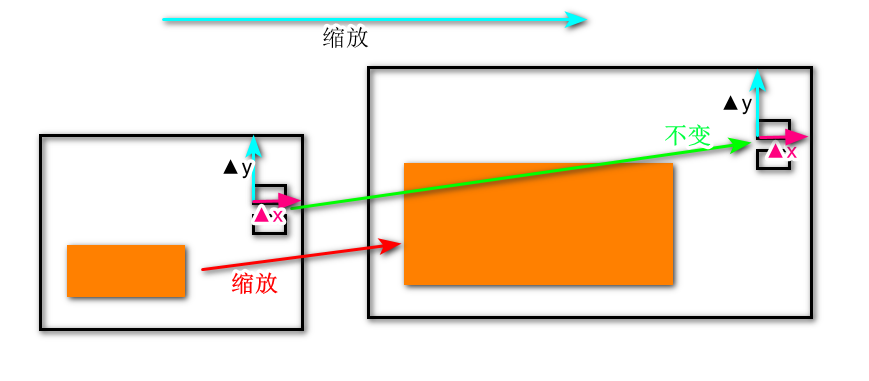如图上示意图，波形尺寸变化，但是要按钮控件保持不变

这就要有一个机制来实现，图形坐标及大小随着figure大小在变动；

根据matlab特性，设计步骤如下：

1.先设置总体可以缩放，在GUI选项中设置，如图1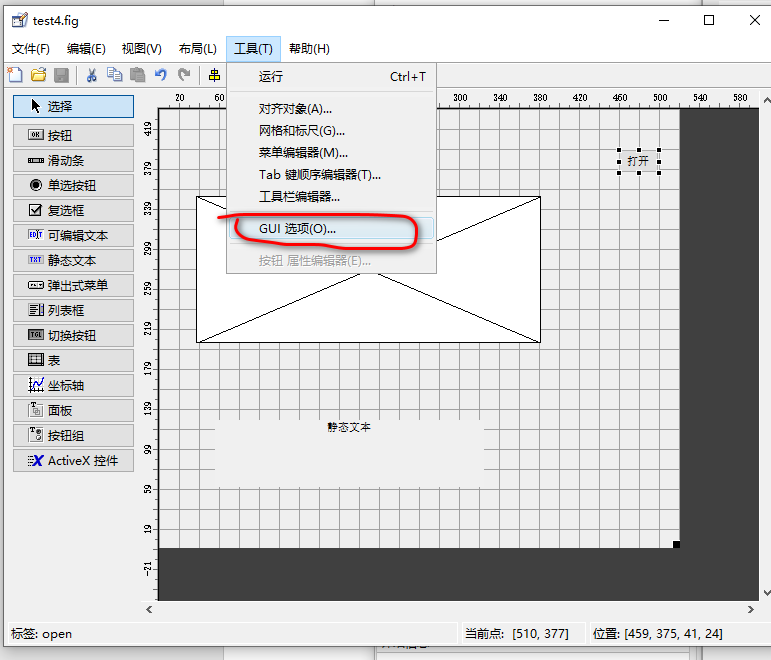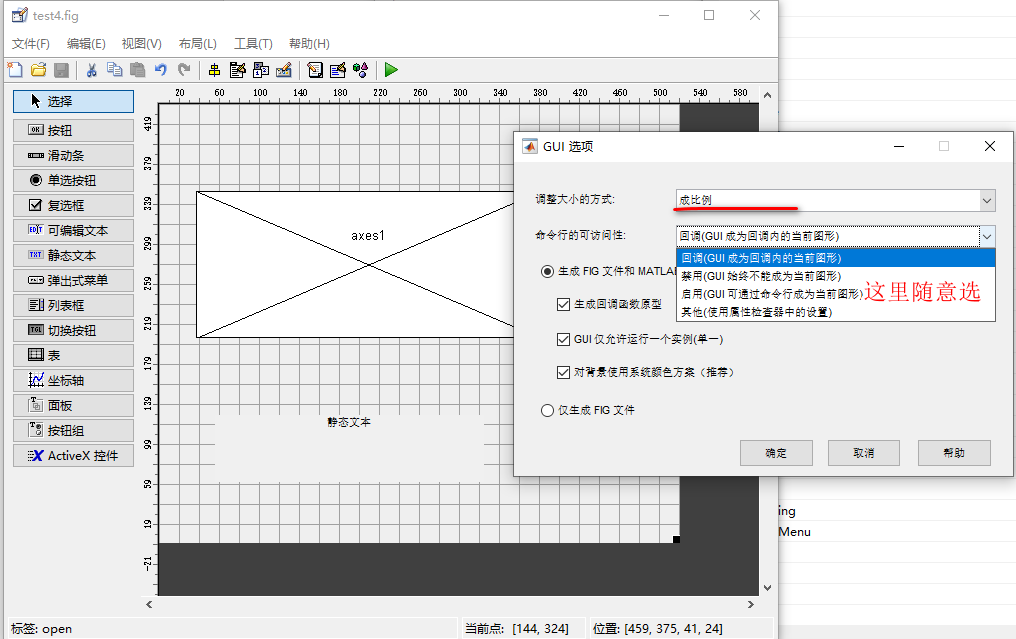图1：gui选项设定

这时整个窗体和所有控件都可缩放，所有控件没有区别

2.单独设置控件不可缩放，在属性中修改Units单位为像素pixels，如图2，这时按钮控件就不能缩放了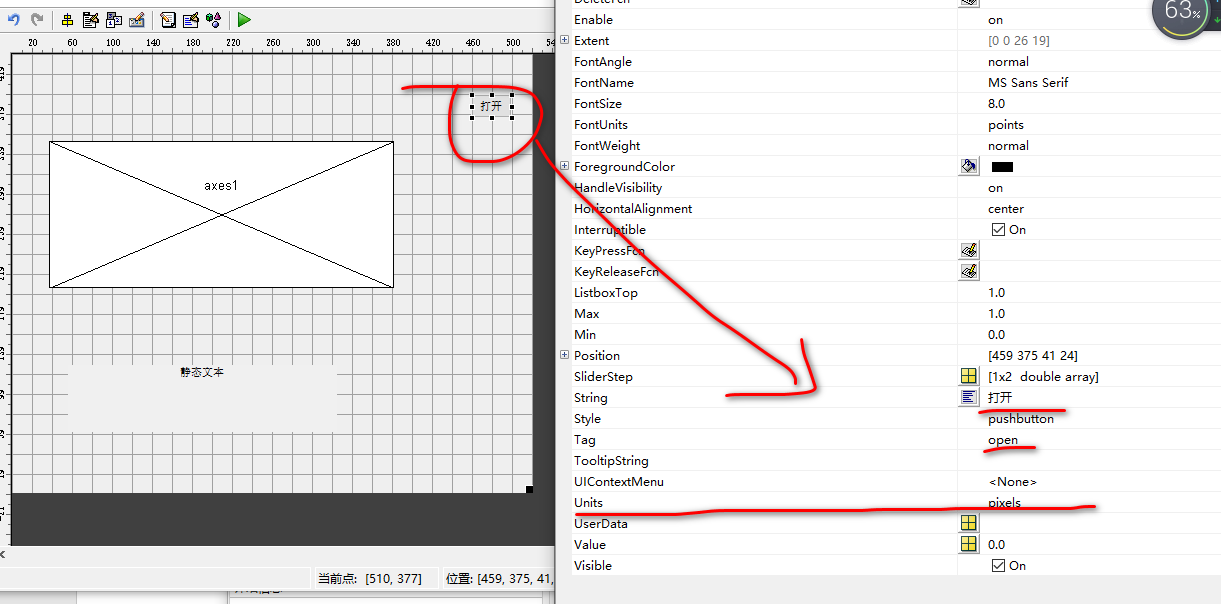图2：按键设定为不能缩放

那可能要问了，这是不是就达到效果了，肯定没有，可以自己试一下；这时会导致控件处在一个固定的位置了，虽然大小不变了，但达不到随着界面大小变化而位置也在随动，如图3；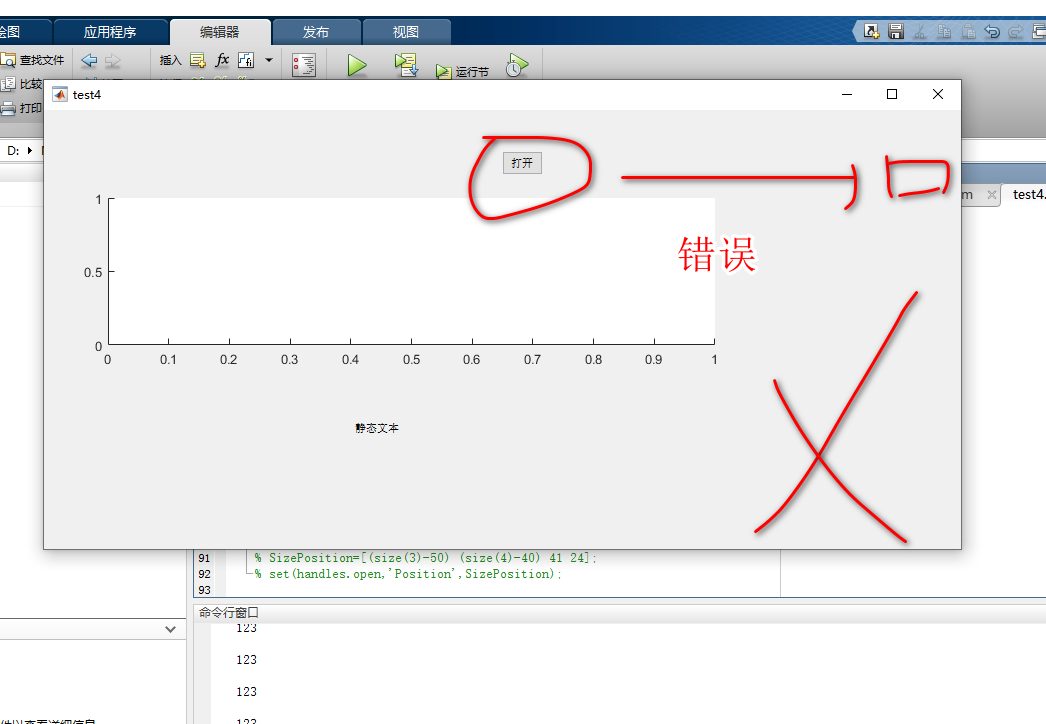图3：错误的效果

3.我们就要解决2中存在的问题

我们观察控件的位置特点，它的位置与fig的右上角的位置差是固定的，所有我们只要读取fig的右上角的坐标后，然后根据xy差值，更新控件的位置信息即可有这个图我们知道只要保证Δx,Δy保持不变就可以使得按钮控件的位置随着fig缩放变化保持一致

另外介绍几点关于坐标的信息：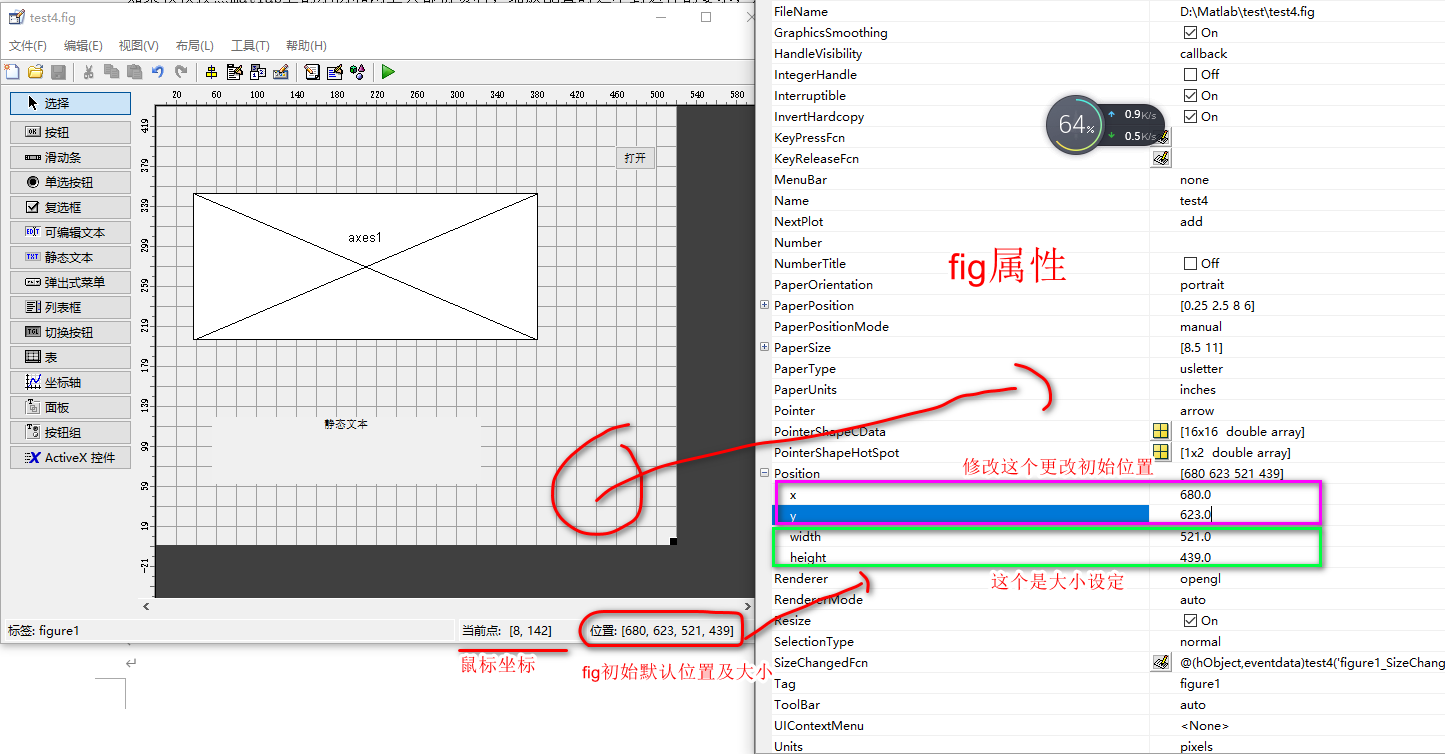在gui设计最低下有坐标显示：当前点：坐标是鼠标所在的位置，位置：是我们选择的控件或fig的位置信息，这里我们选择的是fig，所以就显示的是fig的位置，双击fig弹出属性，看到position是和这个信息一致的；修改position的值就可以改变fig的大小，xy是默认打开fig所在的位置（左下角起点）；当然你选中其他组件，位置显示的是他们对应的信息；

在实际缩放中就是自动改变fig的大小即width、height两个值达到改变fig大小；

现在我们要做的：

第一步：就是读取fig的width、height

首先获取fig的pisition信息

figposition=get(gcf,'position');%这里得到了fig的位置值figposition=[x y  width height ]

figwidth=figposition(3);%宽度，figposition是向量，直接下标引用即可

figheight=figposition(4);%高度

第二步：设定按钮的位置x,y

set(handles.button,'position',[figwidth-Δx  figheight-Δx  width  height ]);

Δx Δy是我们开始设计gui时确定的差值，这个是确定的

4.整体代码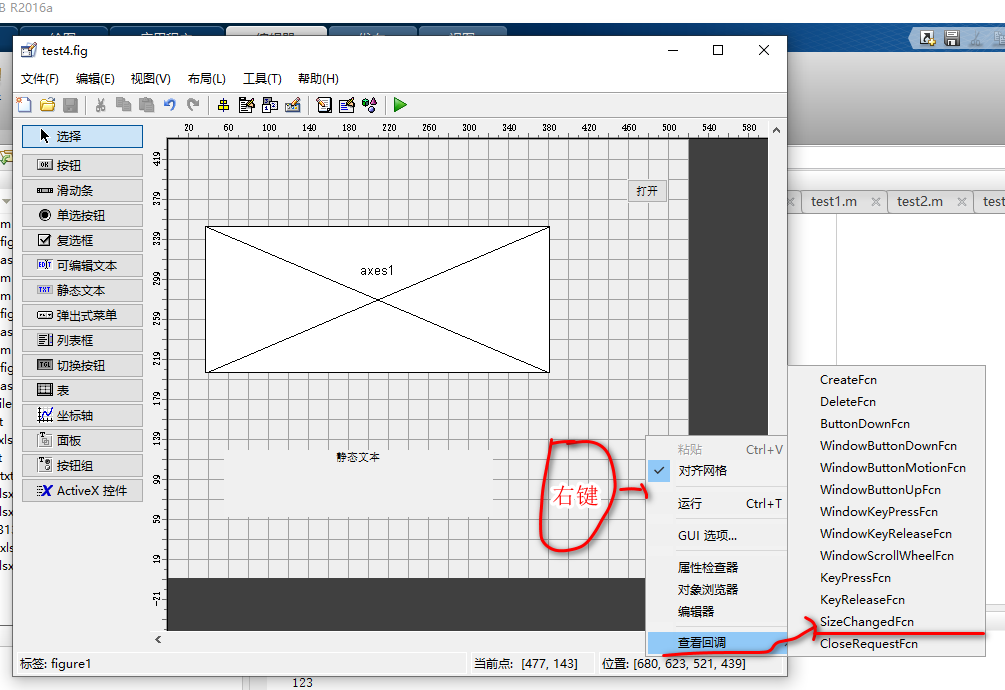这里我们选择在fig的回调函数SizeChangedFcn中来更新按钮的坐标位置

function figure1_SizeChangedFcn(hObject, eventdata, handles)
% hObject    handle to figure1 (see GCBO)
% eventdata  reserved - to be defined in a future version of MATLAB
% handles    structure with handles and user data (see GUIDATA)
size=get(gcf,'Position');
SizePosition=[(size(3)-50) (size(4)-40) 41 24];
set(handles.open,'Position',SizePosition);

5.演示效果：缩放后，按钮保持fig内的位置不变，大小不变；而坐标轴缩放正常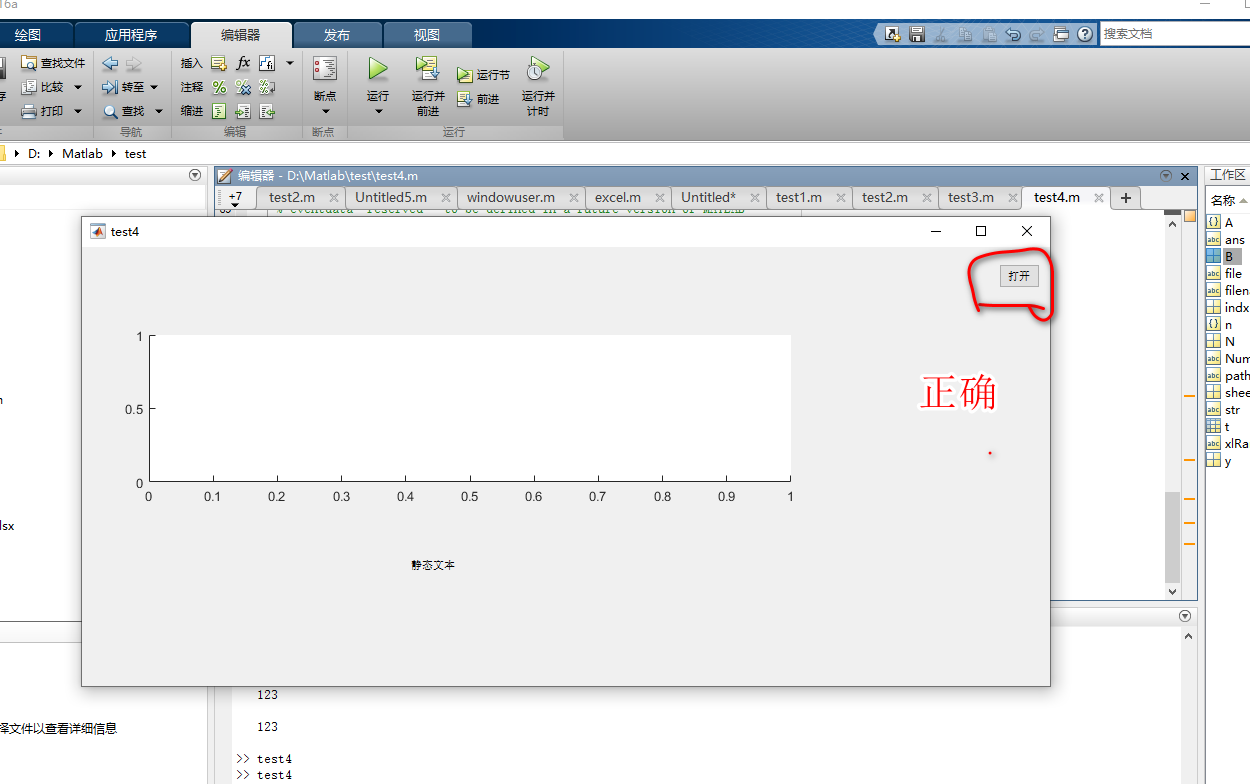这里只以按钮和坐标轴来示例，具体实际那些控件缩放不缩放，由实际需要而定

展开全文matlab gui
• %======用matlab对图像进行缩放(双线性插值法)clear; %此题是用双线性插值法实现图像缩放I=imread('f.jpg');%读入原图像，只需将此处的文件换成要变换的图片即可%图像属性% Filename: 'f.jpg'%FileModDate: '24-Aug-...

%======用matlab对图像进行缩放(双线性插值法)

clear;   %此题是用双线性插值法实现图像缩放

%读入原图像，只需将此处的文件换成要变换的图片即可

%图像属性

%  Filename: 'f.jpg'

%

FileModDate: '24-Aug-2008 16:50:30'

%           FileSize: 20372

%

Format: 'jpg'

%      FormatVersion: ''

%              Width: 480

%

Height: 640

%           BitDepth: 8

%          ColorType:

'grayscale'

%    FormatSignature: ''

%    NumberOfSamples: 1

%

CodingMethod: 'Huffman'

%      CodingProcess: 'Sequential'

%

Comment: {}

[rows,cols]=size(I);

K1 = str2double(inputdlg('请输入行缩放倍数', 'INPUT scale factor', 1,

{'0.5'}));%行默认变为原来的0.5倍

K2 = str2double(inputdlg('请输入列缩放倍数', 'INPUT

scale factor', 1, {'0.4'}));%列默认变为原来的0.4倍

width = K1 * rows;

height = K2 * cols;

Out = uint8(zeros(width,height));  %创建输出图像矩阵

widthScale = rows/width;

heightScale = cols/height;

for x = 6:width - 6           % 6是为了防止矩阵超出边界溢出

for y = 6:height - 6

oldX = x * widthScale;     % oldX,oldY为原坐标，x,y为新坐标

oldY = y * heightScale;

if (oldX/double(uint16(oldX)) == 1.0) &

(oldY/double(uint16(oldY)) == 1.0)

Out(x,y) = I(int16(oldX),int16(oldY));%若oldX，oldY为整数，直接赋值

else

a = double(uint16(oldX));

b = double(uint16(oldY));

x11 = double(I(a,b));                % x11 赋值为 I(a,b)

x12 = double(I(a,b+1));              % x12 赋值为 I(a,b+1)

x21 = double(I(a+1,b));              % x21 赋值为 I(a+1,b)

x22 = double(I(a+1,b+1));            % x22 赋值为

I(a+1,b+1)

Out(x,y) = uint8( (b+1-oldY) * ((oldX-a)*x21 +

(a+1-oldX)*x11) + (oldY-b) * ((oldX-a)*x22 +(a+1-oldX) * x12) );    %

用双线性插值计算公式计算

end

end

end

imshow(I);

figure;

imshow(Out);

%===============使用matlab对图片进行缩放(最近邻域法)

clear;  %此题是用最近邻域法实现图像缩放

%图像属性

%

Filename: 'f.jpg'

%       FileModDate: '24-Aug-2008 16:50:30'

%

FileSize: 20372

%             Format: 'jpg'

%      FormatVersion:

''

%              Width: 480

%             Height: 640

%

BitDepth: 8

%          ColorType: 'grayscale'

%

FormatSignature: ''

%    NumberOfSamples: 1

%       CodingMethod:

'Huffman'

%      CodingProcess: 'Sequential'

%            Comment:

{}

[rows,cols]=size(I);

K1 = str2double(inputdlg('请输入行缩放倍数', 'INPUT scale factor', 1,

{'0.6'}));%行默认变为原来的0.6倍

K2 = str2double(inputdlg('请输入列缩放倍数', 'INPUT

scale factor', 1, {'0.4'}));%列默认变为原来的0.4倍

width = K1 * rows;

height = K2 * cols;

im2 = uint8(zeros(width,height)); %定义输出图像矩阵

widthScale = rows/width;

heightScale = cols/height;

for x = 6:width - 6         %为防止矩阵溢出而选择的参数6

for y = 6:height - 6

oldX = x * widthScale; %oldX，oldY为原坐标，x，y为新坐标

oldY = y * heightScale;

if (oldX/double(uint16(oldX)) == 1.0) &

(oldY/double(uint16(oldY)) == 1.0)

im2(x,y) = I(int16(oldX),int16(oldY));

else

a = double(round(oldX));

b = double(round(oldY)); %若不是整数四舍五入后把临近值赋过去

im2(x,y) = I(a,b);

end

end

end

imshow(I); %输出原图像

figure;

imshow(im2); %输出缩放后图像

%====================用matlab对图像进行旋转(双线性插值法)

clear;%此题是用最近邻域法实现图像旋转

[m,n,p]=size(im1);

%

将图像旋转30度

a=0.5; %a=sin30=0.5

b=0.866;  %b=cos30=0.866

row=n*a+m*b;

col=n*b+m*a;

for

i=1:row                                %先把图象填充成全黑

for j=1:col

im2(i,j,:)=uint8(0);

end

end

for i=1:m                                          %把原图象像素点旋转后变为新图象点

for j=1:n

xx=round(abs((i-m/2)*b-(j-n/2)*a+row/2));

yy=round(abs((i-m/2)*a+(j-n/2)*b+col/2));

for k=1:3

im2(xx,yy,k)=im1(i,j,k);

end

end

end

temp1=uint8(0);

temp2=uint8(0);

temp3=uint8(0);

for i=1:row                                        %把画面上的空点按照最近邻插值法填充

temp1=uint8(0);

temp2=uint8(0);

temp3=uint8(0);

for j=1:col                                    %找到最右的图象边界点

if

(im2(i,j,:)==uint8(0))

else

kk=j;

end

end

for j=1:kk

if (im2(i,j,:)==uint8(0))

im2(i,j,1)=temp1;

im2(i,j,2)=temp2;

im2(i,j,3)=temp3;

else

temp1=im2(i,j,1);

temp2=im2(i,j,2);

temp3=im2(i,j,3);

end

end

end

imshow(im1);

figure;

imwrite(im1,'5.jpg');

%保存原图像

imshow(im2);

imwrite(im2,'6.jpg');%保存旋转后图像

%======================用matlab对图片进行旋转(最近邻域法)

clear;%此题是用最近邻域法实现图像旋转

[m,n,p]=size(im1);

%

将图像旋转30度

a=0.5; %a=sin30=0.5

b=0.866;  %b=cos30=0.866

row=n*a+m*b;

col=n*b+m*a;

for

i=1:row                                %先把图象填充成全黑

for j=1:col

im2(i,j,:)=uint8(0);

end

end

for i=1:m                                          %把原图象像素点旋转后变为新图象点

for j=1:n

xx=round(abs((i-m/2)*b-(j-n/2)*a+row/2));

yy=round(abs((i-m/2)*a+(j-n/2)*b+col/2));

for k=1:3

im2(xx,yy,k)=im1(i,j,k);

end

end

end

temp1=uint8(0);

temp2=uint8(0);

temp3=uint8(0);

for i=1:row                                        %把画面上的空点按照最近邻插值法填充

temp1=uint8(0);

temp2=uint8(0);

temp3=uint8(0);

for j=1:col                                    %找到最右的图象边界点

if

(im2(i,j,:)==uint8(0))

else

kk=j;

end

end

for j=1:kk

if (im2(i,j,:)==uint8(0))

im2(i,j,1)=temp1;

im2(i,j,2)=temp2;

im2(i,j,3)=temp3;

else

temp1=im2(i,j,1);

temp2=im2(i,j,2);

temp3=im2(i,j,3);

end

end

end

imshow(im1);

figure;

imwrite(im1,'5.jpg');

%保存原图像

imshow(im2);

imwrite(im2,'6.jpg');%保存旋转后图像

展开全文• 数字水印 攻击 缩放 matlab代码 缩放的代码 成功率极高
• matlab实现图像的缩放

万次阅读 2019-07-03 19:07:09
matlab实现图像的缩放 缩放同样是仿射变换的一种特例，它接受水平和垂直两个方向的缩放值。 matlab 实现水平，垂直，水平垂直缩放： clear all close all clc img = imread('test.jpg'); %读取输入图片的...

matlab实现图像的缩放

缩放同样是仿射变换的一种特例，它接受水平和垂直两个方向的缩放值。matlab 实现水平，垂直，水平垂直缩放：

clear all
close all
clc
[h,w,d] = size(img); %获取行和列，即原图的高度和宽度
xscale = 3;
yscale = 3;
ht = floor(h./xscale);
wt = floor(w./yscale);
%zoo = zeros(ht,w,d);
for i = 1:ht
for j = 1:w
% for k = 1:d
%zoo(i,j,d) = img(floor(i*xscale),j,d);
% end
zooh(i,j) = img(floor(i*xscale),j);
end
end

for i = 1:h
for j = 1:wt
zoow(i,j) = img(i,floor(j*xscale));
end
end

for i = 1:ht
for j = 1:wt
zoohw(i,j) 
展开全文缩放 matlab实现缩放
• matlab实现图片缩放

千次阅读 2019-07-07 15:38:37
zoomNum为缩放系数 height和width的命名可能反了，不要在意这些细节… I=imread('tissue.png'); figure(1); imshow(I) zoomNum=0.5; [fWidth fHeight]=size(I); fHeight=fHeight/3; cWidth=floor(fWidth*...matlab 图像处理
• Matlab实现图像的比例缩放

万次阅读 多人点赞 2018-05-10 22:35:49
以灰度图像circuit.tif为例，利用Matlab图像处理工具箱中的imresize函数对图像进行比例缩放变换。要求：创建4个figure窗口（不可以用subplot，显示不出来放大效果），分别用于显示原始图像、等比例放大1.5倍后的图像...matlab
• MATLAB实现图形交互式缩放的方法的研究MATLAB实现图形交互式缩放的方法的研究摘要：研究了MATLAB中GUI界面下以鼠标拖放交互方式实现图形缩放问题，分析了不同条件和要求下用MATLAB实现图形缩放的方法和具体算法，...
• 自编Matlab函数实现图像缩放 自编Matlab函数实现图像缩放 自编Matlab函数实现图像缩放 自编Matlab函数实现图像缩放 自编Matlab函数实现图像缩放
• MATLAB:图片缩放

千次阅读 2018-10-21 17:31:33
要求： 将指定文件夹中的所有图片一次缩小后保存，名字与原来的名字相同。 核心语句： S=imresize(rgb,1/6,'nearest'); 整体代码： file_path = 'F:\2018\'; % 图像文件夹路径 img_path_list = dir(strcat(file_...MATLAB
•   首先举个例子说明插值过程，先看看matlab的插值函数 interp() 吧： x = -2 : 1 : 2; y = -2 : 1 : 2; [X, Y] = meshgrid(x, y); Z = -X.^2 - Y.^2; p = -2 : 0.33 : 2; q = -2 : 0.33 : 2; [P, Q] = meshgrid(p,...
• Matlab——图像缩放（插值法）

万次阅读 多人点赞 2018-07-30 15:49:36
用双线性内插法实现位深度为8的灰度图像的缩放。   思路：  输入原图像以及缩放后图像的像素要求（宽度*高度），处理后输出新图像。  我是用matlab来实现scale(input_img,scale_size)函数的，输入图像路径...Matlab 数字图像处理 图像缩放 插值法
• 此外，我想在matlab中更改表面图的外观。数据是二维的(x和y)，x的范围是15000到80000和y从1000到5500。使用线性回归找到第1类和第0类的两条回归线。为此，我缩放了数据，计算了权重，并使用了缩放数据的权重，也对图...matlab模型缩放
• MATLAB图像放大

2015-06-10 21:19:58
基于matlab的图像放大代码，操作简单速度快。图像放大
• Matlab图像缩放 Matlab实现函数代码如下： clc I=rgb2gray(imread('Campus-scenery2sk.JPG')); figure,imshow(I); title('原图'); reduceI=imresize(I,0.5); %原图像I缩小0.5倍 figure,imshow(reduceI); title('...matlab
• 经典图像/视频缩放算法原理及MATLAB\Simulink实现1 图像/视频缩放介绍2 图像缩放的经典算法2.1 最近邻插值法2.2 双线性插值法3 图像缩放算法的MATLAB实现3.1 最近邻算法实现3.2 双线性插值算法实现4 视频无极缩放的...图像处理 缩放
• MATLAB图像分辨率的增强与图像缩放循环重写，本质上是矩阵的数据刷新，重新布局计算。 源码整理如下： % 关闭所有窗口，清理变量，清理屏幕 close all;clear all;clc AI = imread('dfgdg.jpg'); imshow(AI)%显示原图...matlab 计算机视觉 图像识别 机器学习 图像处理
• 启用或禁用缩放或者按比例放大图像 二、详解 1.zoom on zoomon%启用缩放模式。 在图窗中启用缩放模式时，如果在光标处于坐标区内时按下鼠标按键，将会放大或缩小鼠标下面的点。 缩放将会改变坐标区范围。 2....matlab...

matlab缩放matlab 订阅